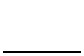Skill 9D
Tens and Ones - Giving Place Values from a Number
We now take place value to its most advanced form which is looking at a number and identifying how many tens and how many ones are in the number.  This requires true understanding of the place value concept.

How many tens and ones are in the numbers shown?

 32 =tensones
 7 =tensones
 19 =tensones